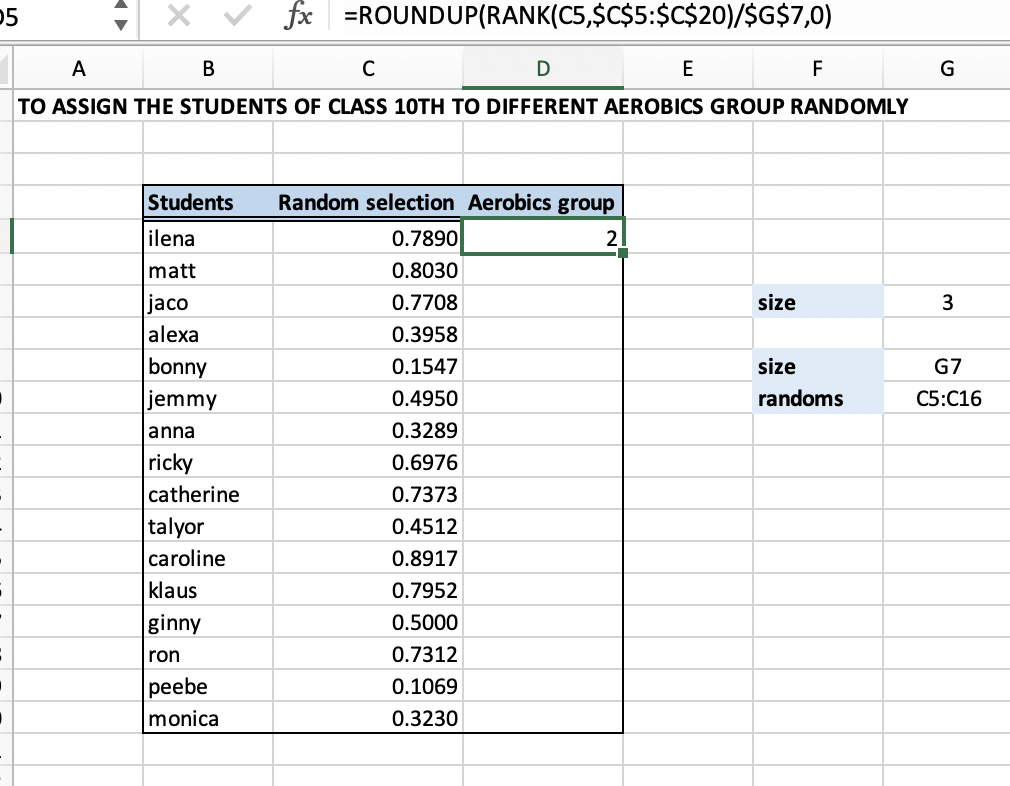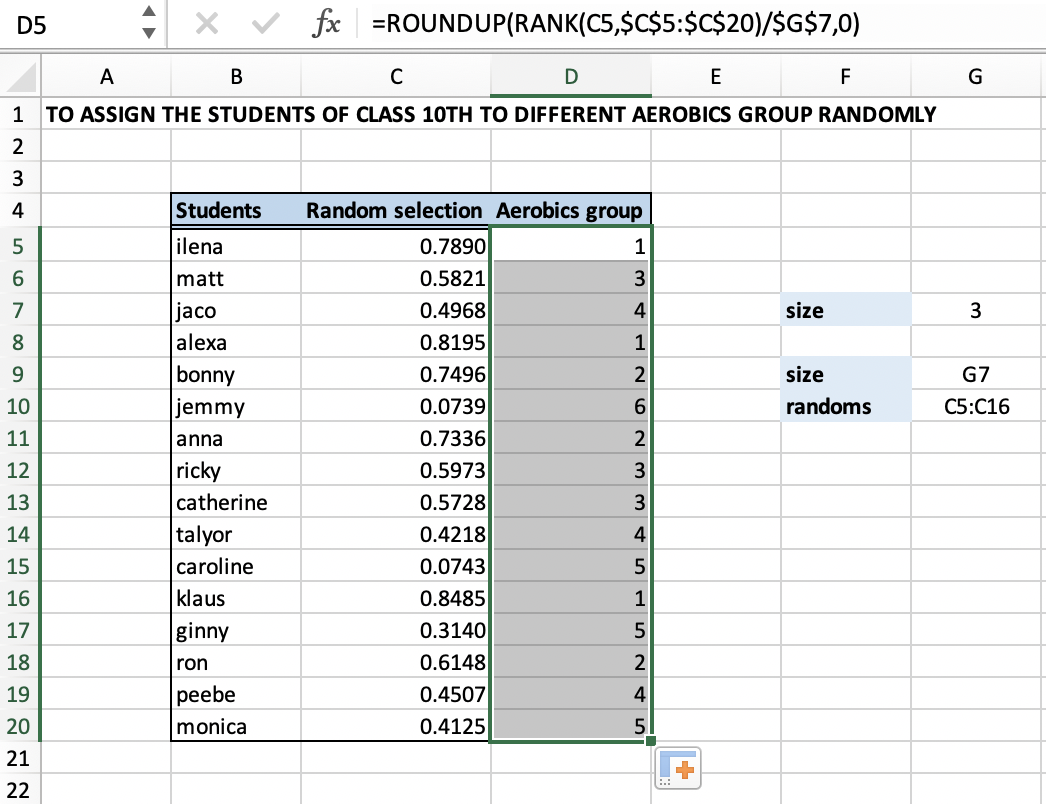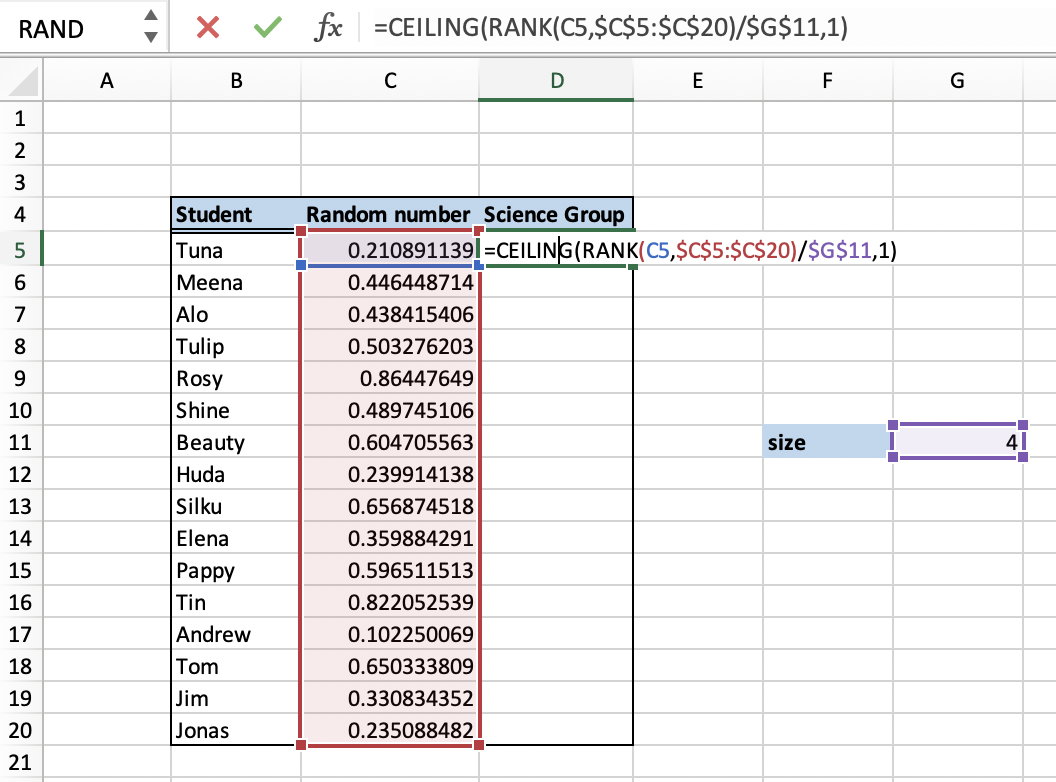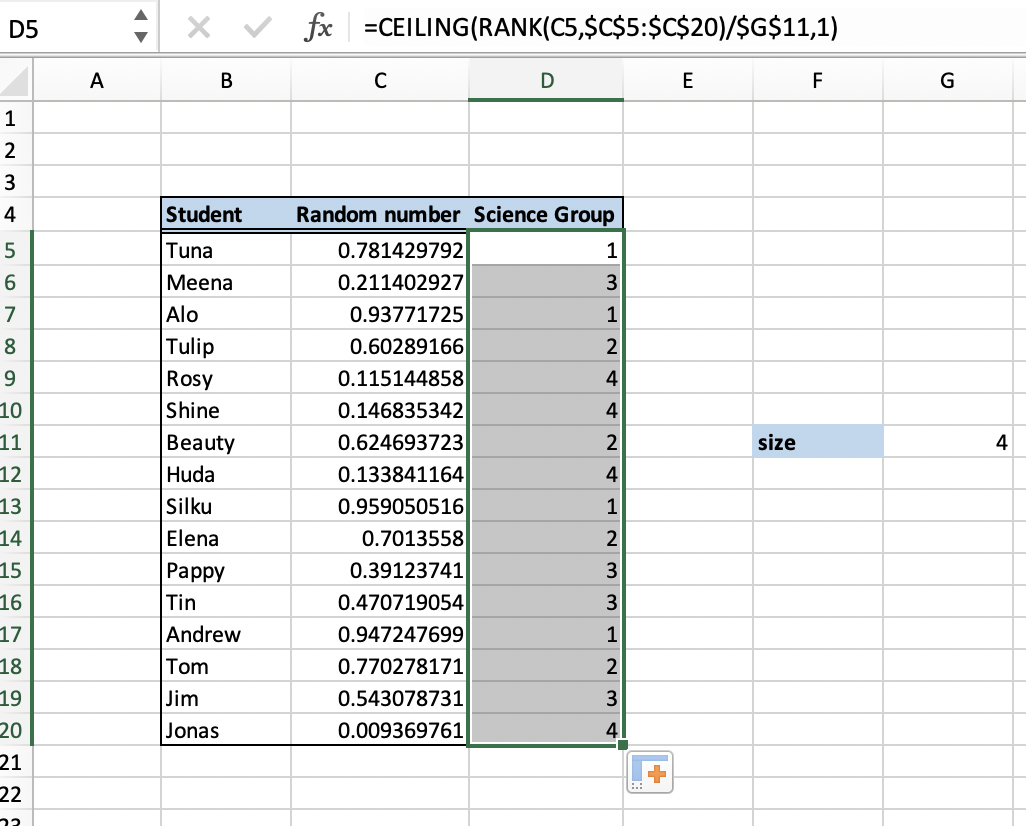Get instant live expert help with Excel or Google Sheets“My Excelchat expert helped me in less than 20 minutes, saving me what would have been 5 hours of work!”

#### Post your problem and you’ll get Expert help in seconds.

Your message must be at least 40 characters
Our professional Expert are available now. Your privacy is guaranteed.

# Randomly Assign People to Groups in Excel

At times we have to randomly assign people to groups. This may seem a mind-numbing task, but it can be made very easy when executed in Excel.

## How to randomly assign people to groups in Excel

Here we can use a formula created by the Excel RANK and ROUNDUP functions together. We also use a helper column where RAND function is used to randomly generate values.

### Formula

To randomly assign people to groups we use the formula

`=ROUNDUP(RANK(A1,randoms)/size,0)`

The above formula returns a group no. for each entry.

Here

randoms’ and ‘size’ are named range.

Random is a helper column generated by Excel RAND Function.

### Explanation of formula

Excel RANK function is an inbuilt function in Excel which returns the rank of a number within a large set of numbers or array. This function is categorized as an inbuilt statistical function in Excel.

Excel ROUNDUP function is another inbuilt function in Excel which returns a number which is rounded up to a definite number of digits. This function rounds away from ‘0’. It is categorized as a Trig or Math function and can be aptly used as a worksheet function in Excel.

### Example

Let us take an example; we have to assign random groups to students for the aerobics class. We provide the size of group 4 in G10 as shown. To create groups, we use the formula shown in D5.

`=ROUNDUP(RANK(C5,random)/size,0)`Figure 1: Illustration of ROUNDUP and RANK formula

Here, “random” is the name of the range [C5:C20] and “size” is the named range[G10].

We can drag the formula and use the above formula for a hefty amount of data.Figure 2: Randomly assign groups

We can also make use of the RAND function, which would generate random numbers. This function will keep on generating random values every time we change the values in the worksheet.

### Alternate Solution

Another approach of assigning random value is by using the CEILING function which is another inbuilt function in Excel.

### Formula

`=CEILING(RANK(C5,random)/size,1)`

### Explanation of formula

This function can be used as an alternative to the ROUNDUP function. The ceiling function rounds up, but instead of rounding to some given number of decimal places, it rounds up to a given multiple.

### Example

As per the example is shown in Figure 1, you can you use the above alternate formula to randomly assign people to groups as shown below.Figure 3: Illustration of CEILING functionFigure 4: Result of applying CEILING function

Still need some help with Excel formatting or have other questions about Excel? Connect with a live Excel expert here for some 1 on 1 help. Your first session is always free.

### Did this post not answer your question? Get a solution from connecting with the expert.Another blog reader asked this question today on Excelchat:
Solution examplesim creating a fantasy hockey model and trying to figure out how to rank if someone has played more than a certain number of games, and if they're certain position how to rank them accordingly
Solved by X. D. in 60 minsI'm Apply a =RANDBETWEEN formula in one cells want to apply Formula to entire column and run formula when i hit the entire on the particular cell
Solved by M. S. in 20 minsHelp! In a coin toss I have a 1 in 2 (fifty-fifty) chance of getting heads or tails. I need a formula to determine my chance of getting 4 heads in a row or 4 tails in a row out of 10 coin tosses. If I get 4 in a row I win. What formula can I use to determine my chances of winning if I repeat the 10 tosses for hundreds or thousands of times? I get as far as =RANDBETWEEN(0,1) but I have not been able to figure out what formula to use to determine if there are 4 in a row in a group of 10 tosses, times thousands of groups with 10 tosses in each group. Thank-you for any help you can provide.
Solved by V. F. in 51 minsI have 4 column. when i fill the first 3 column. in 4th column i need a unique id number and i generate unique id number using RANDBETWEEN formula [ =CHAR(RANDBETWEEN(65,90))&CHAR(RANDBETWEEN(65,90))&RANDBETWEEN(1000,9900) ] but i want ..... when i'm filling the 3 column after that 4th column run this formula and generate unique id automatically
Solved by X. Q. in 20 minsI cannot enter this formula into Excel. I get an error message asking for an apostrophe?IF(\$S2=0,"",IF(\$S2>0,RANK(\$S2,\$S\$2:\$S\$8000),RANK(\$S2,\$S\$2:\$S\$8000-COUNTIF(\$S\$2:\$S\$8000,0)))
Solved by B. W. in 20 mins## Subscribe to Excelchat.coAnother blog reader asked this question today on Excelchat: